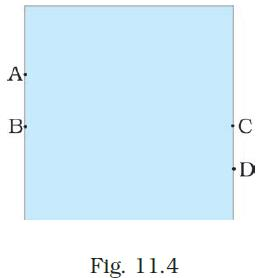# Test: Force And Pressure - 1

## 10 Questions MCQ Test General Science(Prelims) by IRS Divey Sethi | Test: Force And Pressure - 1

Description
This mock test of Test: Force And Pressure - 1 for Class 8 helps you for every Class 8 entrance exam. This contains 10 Multiple Choice Questions for Class 8 Test: Force And Pressure - 1 (mcq) to study with solutions a complete question bank. The solved questions answers in this Test: Force And Pressure - 1 quiz give you a good mix of easy questions and tough questions. Class 8 students definitely take this Test: Force And Pressure - 1 exercise for a better result in the exam. You can find other Test: Force And Pressure - 1 extra questions, long questions & short questions for Class 8 on EduRev as well by searching above.
QUESTION: 1

### A push or pull on an object is called

Solution:

A push or pull on an object is called force. Force can make stationary body in motion and moving body in rest.

QUESTION: 2

### Which one of the following forces is a contact force?

Solution:

Contact forces are those types of forces which result when the two interacting objects are perceived to be physically contacting each other. Examples of contact forces include frictional forces, tensional forces, normal forces, air resistance forces, and applied forces.

QUESTION: 3

### S.I. unit of force is

Solution:

S.I. unit of force is Newton. When a force pushes a body of mass one kg to a distance of one meter, the force is equal to one Newton.

QUESTION: 4

In game of tug-of war, two teams

Solution:

In a game of tug-of war, two teams pull the rope in opposite direction. The team that pulls the rope toward itself will become winner.

QUESTION: 5

Perpendicular force acting per unit area is called

Solution:

The total force acting perpendicular on a given surface is called thrust. Thrust acting upon a unit area is called pressure.

QUESTION: 6

A water tank has four taps fixed at points A, B, C, D as shown in Fig. 11.4. The water will flow out at the same pressure from taps atSolution:

In the given water tank, B and C are at the same levels. Hence, from taps B and C, water will flow out at the same pressure.

QUESTION: 7

S.I. unit of pressure is

Solution:

S.I. unit of pressure is Pascal. One Pascal is equal to one Newton per meter square.

QUESTION: 8

The net force on an object is __________ if the two forces acting on it in opposite directions are equal.

Solution:

The force on an object is zero if the two forces acting on it in opposite directions are equal in magnitude. This kind of force is called balanced force.

QUESTION: 9

A force of 16N acts on an area of 50 cm2. What is the pressure in pascal?

Solution:

F = 16 N,
A = 50 cm2 = 50 / 10000 m2
We know that, P = F / A
∴ P = (16 x 10000) / 50 = 3200 Pa

QUESTION: 10

A force applied on an object may change its

Solution:

A force applied on an object may change its speed. If the force applied on the object is in the direction of its motion, the speed of the object increases. If the force is applied in the direction opposite to the direction of motion, then it results in a decrease in the speed of the object.